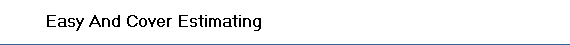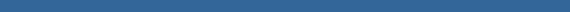##µŃÇÍÂčŇ§ˇŇĂăŞé§ŇąáşşĘ´ : | Beam  | Column | Footing | PierColumn | Slab | Stair | Wall |

### §Ňą Post-Tension Concrete Slab### Post-Tension Concrete Slab

• LeanConcrete = LeanConcreteThickness * 100
• SandFill = SandFillThickness * 100
• Concrete = SlabThickness * 100
• Form = 100
• TopBar = 100 / TopBar * 2 * (1 + LappedTopBar)* WeighOfBar
• BottomBar = 100 / BottomBar * 2 * (1 + LappedBottomBar)* WeighOfBar
• Wire = TotalBar*ConstantWire/1000
• Nail = Form*ConStantNail### Post-Tension Concrete Beam

• Concrete = (Width * Depth - Width * SlabThickness) * (Length - ColumnWidth)
• Form = (Depth - SlabThickness) * 2 * (Length - ColumnWidth)
• MainBar1 = Length * MainBar1 * (1 + LappedMainBar)* WeighOfBar
• MainBar2 = Length * MainBar2 * (1 + LappedMainBar)* WeighOfBar
• StirrupBar = Length / StirrupBar * LengthStirrupBar * WeighOfBar
• Wire = TotalBar*ConstantWire/1000
• Nail = Form*ConStantNail### Column Capital

• Concrete = Width * Length * (Thickness - SlabThickness)
• Form = 2 * (Width + Length) * (Thickness - SlabThickness)
• LengthBar = (Length + 2 * Thickness) * LengthBar * WeighOfBar
• WidthBar = (Width + 2 * Thickness) * WidthBar * WeighOfBar
• StirrupBar = 2 * (Length + Width) * StirrupBar * WeighOfBar
• Wire = TotalBar*ConstantWire/1000
• Nail = Form*ConStantNail### Shear Stirrup

• MainBar = Length * MainBar * WeighOfBar
• StirrupBar = Length / StirrupBar * (Width + SlabThickness) * 2 * WeighOfBar### Pour Strip

• Concrete = Width * Thickness * Length
• Form = Width * Length
• HorizontalBar = Width / HorizontalBar * Length * 2 * (1 + LappedHorizontalBar) *WeighOfBar
• LeftExtraBarB = Length / LeftExtraBarB * LengthLeftExtraBarB * WeighOfBar
• LeftExtraBarT = Length / LeftExtraBarT * LengthLeftExtraBarT * WeighOfBar
• RightExtraBarB = Length / RightExtraBarB * LengthRightExtraBarB * WeighOfBar
• RightExtraBarT = Length / RightExtraBarT * LengthRightExtraBarT * WeighOfBar### Top Reinforcement (T)

• Rebar = ReBar * LengthReBar * WeighOfBar### Top & Bottom Reinforcement (R)

• BottomRebar = 100 / BottomReBar * 2 * (1 + LappedReBar) * WeighOfBar
• TopRebar = 100 / TopReBar * 2 * (1 + LappedReBar) * WeighOfBar### Reinforcement at Anchorage of Uniform Tender

• MainBar = Length * MainBar * (1 + LappedMainBar)* WeighOfBar
• StirrupBar = Length / StirrupBar * (Width + Depth) * 2 * WeighOfBar### Reinforcement at Anchorage of Banded Tender

• MainBar = Length * MainBar * WeighOfBar
• StirrupBar = Length / StirrupBar * (Width + Depth) * 2 * WeighOfBar### Reinforcement at Skew Edge

• Rebar1 = 2 * (LengthOfRebar + (Width2 + Thickness) / 2) * Width1 / ReBar1 * WeighOfBar
• Rebar2 = 2 * (LengthOfRebar + (Width1 + Thickness) / 2) * Width2 / ReBar2 * WeighOfBar### Reinforcement at PC. Slab-Basement Wall Joint

• HorizontalBar1 = HorizontalBar1 * 12 * (1 + LappedHorizontalBar) * WeighOfBar
• HorizontalBar2 = HorizontalBar2 * 12 * (1 + LappedHorizontalBar) * WeighOfBar
• UBar = (UpperLengthUbar + LowerLengthUbar + SlabThickness + 2 * WallThickness) * 12 / UBar * WeighOfBar
• StirrupBar = 2 * (SlabThickness + WallThickness) * 12 / StirrupBar * WeighOfBar### Reinforcement at Concrete Joint

• Rebar1 = 2 * Width * 12 / ReBar1 * WeighOfBar
• Rebar2 = 24 * Width / ReBar2 * (1 + LappedOfRebar2) * WeighOfBar### Reinforcement at Exterior Beam

• BottomBar1 = (HookBottomBar1 + LengthBottomBar1) * 12 / BottomBar1 * WeighOfBar
• BottomBar2 = 12 * BottomBar2 * (1 + LappedBottomBar2) * WeighOfBar
• TopBar1 = (HookTopBar1 + LengthTopBar1) * 12 / TopBar1 * WeighOfBar
• TopBar2 = 12 * TopBar2 * (1 + LappedTopBar2) * WeighOfBar### Reinforcement at Interior Beam

• Rebar1 = LengthReBar1 * 12 / ReBar1 * WeighOfBar
• Rebar2 = 12 * ReBar2 * (1 + LappedReBar2) * WeighOfBar
Reinforcement for Drop Elevation
• ExtraBar = 2 * 12 / ExtraBar * LengthExtraBar * WeighOfBar
• Rebar = 12 * ReBar * (1 + LappedReBar) * WeighOfBar
• StirrupBar = LengthStirrupBar * 12 / StirrupBar * WeighOfBar### Reinforcement for Opening

• Rebar1 = 2 * LengthReBar1 * ReBar1 * WeighOfBar
• Rebar2 = 2 * LengthReBar2 * ReBar2 * WeighOfBar
• Rebar3 = 2 * LengthReBar3 * ReBar3 * WeighOfBar
• StirrupBar1 = LengthStirrupBar1 * 2 * LengthReBar1 / StirrupBar1 * WeighOfBar
• StirrupBar2 = LengthStirrupBar2 * 2 * LengthReBar2 / StirrupBar2 * WeighOfBar### Reinforcement for Corner of Slab

• Rebar1 = 2 * LengthReBar1 * ReBar1 * WeighOfBar
• Rebar2 = LengthReBar2 * ReBar2 * WeighOfBar